Courses

# Important Formulae for Calorimetry and Heat Transfer NEET Notes | EduRev

## JEE : Important Formulae for Calorimetry and Heat Transfer NEET Notes | EduRev

The document Important Formulae for Calorimetry and Heat Transfer NEET Notes | EduRev is a part of the JEE Course DC Pandey Solutions for JEE Physics.
All you need of JEE at this link: JEE

1. Calorimetry

(i) Q = msΔθ = cΔθ, when temperature changes without change in state.
(ii) Q = mL, when state changes without change in temperature.
(iii) s = specific heat of any substance
= heat required to increase the temperature of unit mass by 1°C or 1 K.
(iv) c = heat capacity of a body = ms
= heat required to increase the temperature of whole body by 1°C or 1 K.
(v) Specific heat of water is 1 cal g-1 - °C between, 14.5°C and 15.5°C.

(vi) L = latent heat of any substance
= heat required to convert unit mass of that substance from one state to another state.
(vii) Water equivalent of a vessel It is mass of equivalent water which takes same amount of heat as taken by the vessel for same rise of temperature.
2. Heat Transfer
Heat conduction through a rod
(i) Heat flow in steady state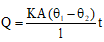(ii) Rate of flow of heat = heat current or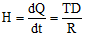Here, TD = temperature difference = θ1 - θ2 and R = thermal resistance = 1/KA
(i) Absorptive power, a=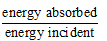a ≤ 1
a = 1 for perfectly black body.
(ii) Spectral absorptive power al = absorptive power of wavelength l.
al ≤ 1
al = 1 for perfectly black body.
(iii) Emissive power e Energy radiated per unit area per unit time is called emissive power of a body. Its SI units are Js-1m-2 or Wm-2.
(iv) Spectral emissive power el Emissive power of wavelength l is known as spectral emissive power.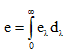(v) Stefan's law Emissive power of a body is given by,
e = erσT4
Here er = emissivity, emittance, relative emissivity or relative emittance.
er ≤ 1
er = 1 for a perfectly black body.
Sometimes emissivity is also denoted bye. In that case differentiate them by their units. er is unitless while e has the units Wm-2.
(vi) Total energy radiated by a body,
E = erσT4 At
Here, A = surface area and t = time.
(vii) a = er or absorptivity of a body = its emissivity.
(viii) Kirchhoff s law If different bodies (including a perfectly black body) are kept at same temperatures, then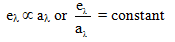or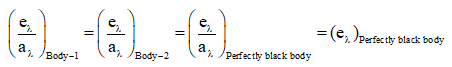From this law following two conclusions can be drawn.
(a) Good absorbers of a particular wavelengthare also good emitters of same wavelength l.(b) At a given temperature, ratio of ql and al for any body is constant. This ratio is equal to el of perfectly black body at that temperature.
(ix) Wien's displacement law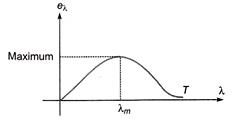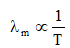or
λmT = Constant
= Wien's constant b Here, b = 2.89 × 10-3 m-K

Further, area of this graph will give total emissive power which is proportional to T4,

(x) Cooling of a body by radiation

(a) Rate of cooling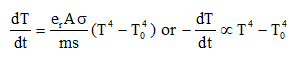(b) Newton's law of cooling If temperature difference of a body with atmosphere is small, then rate of cooling µ temperature difference.
(c) If body cools by radiation according to Newton, then temperature of body decreases exponentially.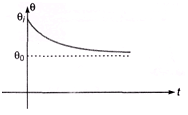In the figure,

θI = initial temperature of body

θ0 = temperature of atmosphere.
Temperature at any time t can be written as, θ = θ0 + (θI - θ0)e-αt
(d) If body is cooling according to Newton then to find temperature of a body at any time t, we will have to calculate e-αt. To avoid this, you can use a shortcut approximate formula given below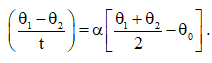Offer running on EduRev: Apply code STAYHOME200 to get INR 200 off on our premium plan EduRev Infinity!

210 docs

,

,

,

,

,

,

,

,

,

,

,

,

,

,

,

,

,

,

,

,

,

;